# partition

## Dividing up the unrestricted partitions ★★

Author(s): David S.; Newman

Begin with the generating function for unrestricted partitions:

(1+x+x^2+...)(1+x^2+x^4+...)(1+x^3+x^6+...)...

Now change some of the plus signs to minus signs. The resulting series will have coefficients congruent, mod 2, to the coefficients of the generating series for unrestricted partitions. I conjecture that the signs may be chosen such that all the coefficients of the series are either 1, -1, or zero.

Keywords: congruence properties; partition

## Friendly partitions ★★

Author(s): DeVos

A friendly partition of a graph is a partition of the vertices into two sets so that every vertex has at least as many neighbours in its own class as in the other.

Problem   Is it true that for every, all but finitely many-regular graphs have friendly partitions?

Keywords: edge-cut; partition; regular

## Unfriendly partitions ★★★

Author(s): Cowan; Emerson

Ifis a graph, we say that a partition ofis unfriendly if every vertex has at least as many neighbors in the other classes as in its own.

Problem   Does every countably infinite graph have an unfriendly partition into two sets?

Keywords: coloring; infinite graph; partition

## Aharoni-Berger conjecture ★★★

Author(s): Aharoni; Berger

Conjecture   If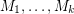are matroids on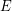andfor every partition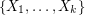of, then there existswithwhich is independent in every.

Keywords: independent set; matroid; partition

## Bounded colorings for planar graphs ★★

Author(s): Alon; Ding; Oporowski; Vertigan

Question   Does there exists a fixed functionso that every planar graph of maximum degreehas a partition of its vertex set into at most three setsso that for, every component of the graph induced by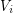has size at most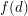?

Keywords: coloring; partition; planar graph

## Linial-Berge path partition duality ★★★

Author(s): Berge; Linial

Conjecture   The minimum-norm of a path partition on a directed graph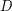is no more than the maximal size of an induced-colorable subgraph.

Keywords: coloring; directed path; partition